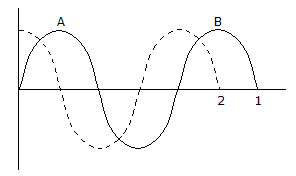# Civil Engineering - Elements of Remote Sensing

Exercise : Elements of Remote Sensing - Section 1
26.
The value of energy quantum for radiation of any frequency is proportional to :
the frequency
the reciprocal of the energy
the square of the frequency
the square root of the frequency
Explanation:
No answer description is available. Let's discuss.

27.
The phase difference of the waves 1 and 2 at A in the given figure is :π/4
π/2
π
3π/2
Explanation:
No answer description is available. Let's discuss.

28.
A perfectly black body :
is a diffuse emitter
absorbs all the radiations of every wave lengths
emits power of every wave length
All the above
Explanation:
No answer description is available. Let's discuss.

29.
Pick up the correct statement from the following :
When the electric field oscillates in the direction of the electric vector, a plane polarised wave is formed.
When the electric vector is in the plane of incidence, vertical polarisation is formed.
When the electric vector is at right angles to the plane of incidence, horizontal polarization wave is formed.
The plane containing the incident ray and normal to the reflecting surface at the point of incidence is called the plane of incidence.
All of these